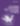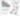# Making Excel The CarlosAg Way

Programming

Share:

#### Free JavaScript Book!Write powerful, clean and maintainable JavaScript.

RRP \$11.95

One question we see a lot on the Sitepoint ASP.NET forums is “Hey, my boss needs me to make an Excel spreadsheet from this here data set. Please help. You are my last hope before I get fired!”

Now, there are a number of ways to generate an excel spreadsheet. The obvious way it to use COM interop and Excel’s own object model to do the job. This is possibly the worst plan. First, it requires one install Excel on the web server to work. Second, Excel is designed to run in user mode, and will pop modial dialog boxes—effectively freezing your applications. I shall ignore the “fun” tasks associated with COM interop and the pure joy of dealing with the Excel object model. Nevermind scalability issues and the like.

The second set of obvious options can get a bit expensive—the various commercial excel generation libraries. Another option in this vein—and possibly the most useful one—is Sql Server 2005 Reporting Services. But only if you are already using it for other reporting tasks, implementing it for some excel export alone is a bit of a waste. The other old standby is to generate a html table and tell a browser "yes, by jove, this is excel." But this is a limited solution at best. What is a developer to do?

Enter CarlosAg

But there is one other option. Modern versions [2002/XP or later] of Excel support the Xml Spreasheet specification. And if .NET is good for just one thing, it would beXml. Enter CarlosAg’s ExcelXmlWriter library. I have used it on a few projects and I must say it is very slick. It is the only free library I have seen which allow a developer to create a nearly fully-featured spreadsheet—including formulas, heavily styled cells and pivot tables—without crossing the COM bridge or paying significant cash.

Now, its not without its issues. The most significant one is that the developer must be very careful to properly format data because is no type filtering at the library level. Just like in any export operation, one must test the output carefully to make certain it is parsable. Nor is there a native .NET 2.0 binary available as of this writing. But I have not yet ran into a show-stopping bug. It is definitely a solid library and clearly quite sweet for the price—free.

And now we get to the good part—code samples. Lets say you need to, oh, just dump a dataset into Carlos’ writer for posterity’s sake.

Well, first you need a class to deal with the dataset as a whole:

``````
using System.Data;
using CarlosAg.ExcelXmlWriter;

namespace WWB.ExcelDatasetWriter
{
/// <summary>
/// Creates an excel-friendly Xml Document from a dataset using the CarlosAg.ExcelXmlWriter library.
/// </summary>

public class ExcelDatasetWriter
{
public ExcelDatasetWriter()
{}

public Workbook CreateWorkbook(DataSet data)
{
//ensure valid data
if (data==null)
{
throw new ArgumentNullException("data", "Data cannot be null.");
}
ensureTables(data);

//Variable declarations
//our workbook
Workbook wb=new Workbook();
//Our worksheet container
Worksheet ws;
//Our DataTableWriter
ExcelDataTableWriter edtw=new ExcelDataTableWriter();
//Our sheet name
string wsName;
//Our counter
int tCnt=0;

//Loop through datatables and create worksheets
foreach (DataTable dt in data.Tables)
{
//set the name of the worksheet
if (dt.TableName!=null && dt.TableName.Length>0 && dt.TableName!="Table")
{
wsName=dt.TableName;
}
else
{
//Go to generic Sheet1 . . . SheetN
wsName="Sheet" + (tCnt+1).ToString();
}
//Instantiate the worksheet
//Populate the worksheet
edtw.PopulateWorksheet(dt, ws);
tCnt++;
}
return wb;
}

private void ensureTables(DataSet data)
{
if (data.Tables.Count==0)
{
throw new ArgumentOutOfRangeException("data", "DataSet does not contain any tables.");
}
}
}
}
``````

Then you need a class to deal with each of the DataTables

``````
using System;
using System.Data;
using CarlosAg.ExcelXmlWriter;

namespace WWB.ExcelDatasetWriter
{
/// <summary>
/// Writes a datatable to a worksheet using the CarlosAG.ExcelXmlWriter library.
/// </summary>

public class ExcelDataTableWriter
{
public ExcelDataTableWriter()
{}

public void PopulateWorksheet(DataTable dt, Worksheet toPopulate)
{
PopulateWorksheet(dt, toPopulate, true);
}

public void PopulateWorksheet(DataTable dt, Worksheet toPopulate, bool makeHeader)
{
//check valid input
if (toPopulate==null)
{
throw new ArgumentNullException("toPopulate", "Worksheet cannot be null.");
}
if (dt==null)
{
throw new ArgumentNullException("dt", "DataTable cannot be null");
}
//Parse the columns
ColumnType[] colDesc=parseColumns(dt);
{
}
//Create rows
foreach (DataRow row in dt.Rows)
{
}
}

#region row + cell making

{
WorksheetRow ret=new WorksheetRow();
foreach(ColumnType ctd in cols)
{
}
return ret;
}

private WorksheetRow makeDataRow(ColumnType[] ctds, DataRow row)
{
WorksheetRow ret=new WorksheetRow();
WorksheetCell tmp=null;
for (int i=0; i<row.Table.Columns.Count; i++)
{
tmp=ctds[i].GetDataCell(row[i]);
}
return ret;
}

#endregion

#region column parsing
private ColumnType[] parseColumns(DataTable dt)
{
ColumnType[] ret=new ColumnType[dt.Columns.Count];
ColumnType ctd=null;
for (int i=0; i<dt.Columns.Count; i++)
{
ctd=new ColumnType();
ctd.Name=dt.Columns[i].ColumnName;
getDataType(dt.Columns[i], ctd);
ret[i]=ctd;
}
return ret;
}

private void getDataType(DataColumn col, ColumnType desc)
{
if (col.DataType==typeof(DateTime))
{
desc.ExcelType=DataType.DateTime;
}
else if (col.DataType==typeof(string))
{
desc.ExcelType=DataType.String;
}
else if (col.DataType==typeof(sbyte)
|| col.DataType==typeof(byte)
|| col.DataType==typeof(short)
|| col.DataType==typeof(ushort)
|| col.DataType==typeof(int)
|| col.DataType==typeof(uint)
|| col.DataType==typeof(long)
|| col.DataType==typeof(ulong)
|| col.DataType==typeof(float)
|| col.DataType==typeof(double)
|| col.DataType==typeof(decimal)
)
{
desc.ExcelType=DataType.Number;
}
else
{
desc.ExcelType=DataType.String;
}
}
#endregion
}
}
```
```

And finally, this class rests on a class to deal with the individual column types.

``````
using System;
using CarlosAg.ExcelXmlWriter;

namespace WWB.ExcelDatasetWriter
{
/// <summary>
/// Creates a Column for CarlosAg.ExcelXmlWriter
/// </summary>

internal class ColumnType
{
public ColumnType()
{}
private string name;
public string Name
{
get {return name;}
set {name=value;}
}

private DataType excelType;
public DataType ExcelType
{
get {return excelType;}
set {excelType=value;}
}

{
}

private string getDataTypeFormatString()
{
if (ExcelType==DataType.DateTime)
{
return "s";
}
return null;
}

public WorksheetCell GetDataCell(object data)
{
WorksheetCell dc=new WorksheetCell();
dc.Data.Type=ExcelType;
if (ExcelType==DataType.DateTime && data is DateTime)
{
DateTime dt=(DateTime)data;
dc.Data.Text=dt.ToString("s");
}
else
{
string dataString=data.ToString();
if (dataString == null || dataString.Length==0)
{
dc.Data.Type=DataType.String;
dc.Data.Text=string.Empty;
}
else
{
dc.Data.Text=dataString;
}
}
return dc;
}
}
}
```
```

Now, the first two code samples are quite straight-forwards. But the final one deals with a few quirks of the library. The main issue is the WorksheetCell.Data.Text property is actually a string, not an object. It literally writes its value to the spreadsheet. And sometimes this can conflict with the DataType property, leading to interesting (and hard to pinpoint) excel errors. In any case, the GetCellData(object) method wraps reasonably effectively for general purpose use.

Now, to make excel open the spreadsheet, you just need to remember to stream it back to the browser with the appropriate content type:

``````
ExcelDatasetWriter edw=new ExcelDatasetWriter();
Workbook wb=CreateWorksheet(MyDataSet);
Response.ContentType = "application/vnd.ms-excel";
wb.Save(Response.OutputStream);
```
```

So, to summarize:

1. Writing excel is a common need.
2. Using Excel object libraries on a web server is a bad idea.
3. Writing Excel using managed code is a good idea.
4. CarlosAg’s excel writer helps you generate excel without ever stepping into interop land.

Enjoy and happy coding.

### Related Articles### Wyatt Barnett

#### New books out now!Learn the basics of programming with the web's most popular language - JavaScript